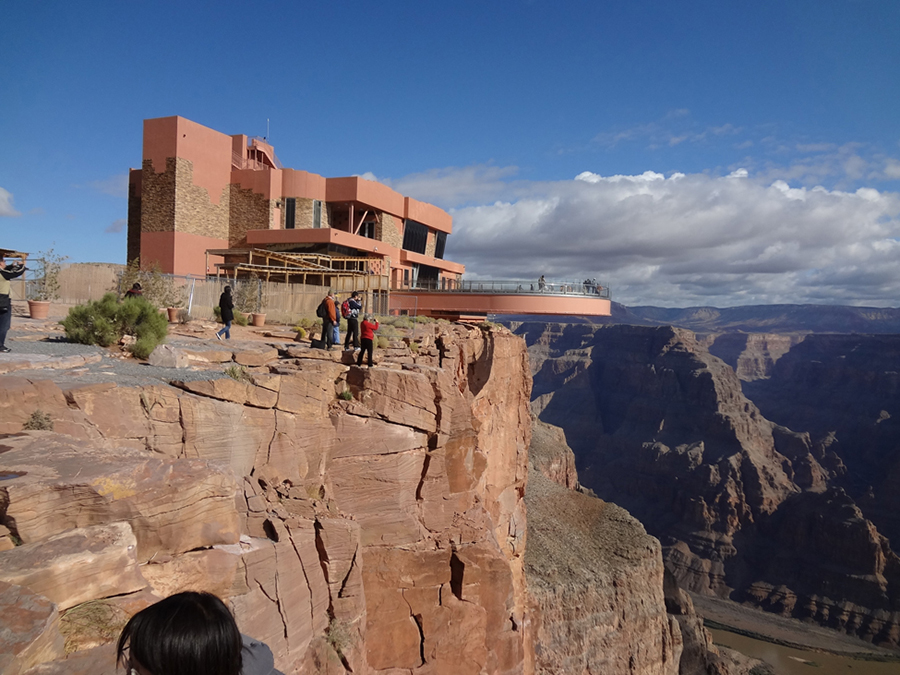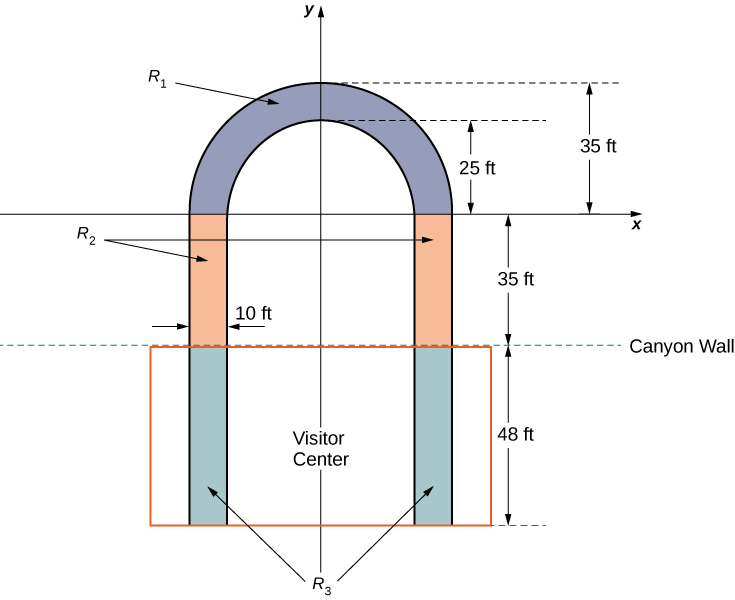## Symmetry and Centroids

### Learning Outcomes

• Apply the theorem of Pappus for volume.

## The Symmetry Principle

We stated the symmetry principle earlier, when we were looking at the centroid of a rectangle. The symmetry principle can be a great help when finding centroids of regions that are symmetric. Consider the following example.

### Example: Finding the Centroid of a Symmetric Region

Let R be the region bounded above by the graph of the function $f(x)=4-{x}^{2}$ and below by the $x$-axis. Find the centroid of the region.

Watch the following video to see the worked solution to Example: Finding the Centroid of a Symmetric Region.

### Try It

Let R be the region bounded above by the graph of the function $f(x)=1-{x}^{2}$ and below by $x$-axis. Find the centroid of the region.

### Activity: Engineering The Grand Canyon Skywalk

The Grand Canyon Skywalk opened to the public on March 28, 2007. This engineering marvel is a horseshoe-shaped observation platform suspended 4000 ft above the Colorado River on the West Rim of the Grand Canyon. Its crystal-clear glass floor allows stunning views of the canyon below (see the following figure).Figure 11. The Grand Canyon Skywalk offers magnificent views of the canyon. (credit: 10da_ralta, Wikimedia Commons)

The Skywalk is a cantilever design, meaning that the observation platform extends over the rim of the canyon, with no visible means of support below it. Despite the lack of visible support posts or struts, cantilever structures are engineered to be very stable and the Skywalk is no exception. The observation platform is attached firmly to support posts that extend 46 ft down into bedrock. The structure was built to withstand 100-mph winds and an 8.0-magnitude earthquake within 50 mi, and is capable of supporting more than 70,000,000 lb.

One factor affecting the stability of the Skywalk is the center of gravity of the structure. We are going to calculate the center of gravity of the Skywalk, and examine how the center of gravity changes when tourists walk out onto the observation platform.

The observation platform is U-shaped. The legs of the U are 10 ft wide and begin on land, under the visitors’ center, 48 ft from the edge of the canyon. The platform extends 70 ft over the edge of the canyon.

To calculate the center of mass of the structure, we treat it as a lamina and use a two-dimensional region in the xy-plane to represent the platform. We begin by dividing the region into three subregions so we can consider each subregion separately. The first region, denoted ${R}_{1},$ consists of the curved part of the U. We model ${R}_{1}$ as a semicircular annulus, with inner radius 25 ft and outer radius 35 ft, centered at the origin (see the following figure).Figure 12. We model the Skywalk with three sub-regions.

The legs of the platform, extending 35 ft between ${R}_{1}$ and the canyon wall, comprise the second sub-region, ${R}_{2}.$ Last, the ends of the legs, which extend 48 ft under the visitor center, comprise the third sub-region, ${R}_{3}.$ Assume the density of the lamina is constant and assume the total weight of the platform is 1,200,000 lb (not including the weight of the visitor center; we will consider that later). Use $g=32{\text{ft/sec}}^{2}.$

1. Compute the area of each of the three sub-regions. Note that the areas of regions ${R}_{2}$ and ${R}_{3}$ should include the areas of the legs only, not the open space between them. Round answers to the nearest square foot.
2. Determine the mass associated with each of the three sub-regions.
3. Calculate the center of mass of each of the three sub-regions.
4. Now, treat each of the three sub-regions as a point mass located at the center of mass of the corresponding sub-region. Using this representation, calculate the center of mass of the entire platform.
5. Assume the visitor center weighs 2,200,000 lb, with a center of mass corresponding to the center of mass of ${R}_{3}.$ Treating the visitor center as a point mass, recalculate the center of mass of the system. How does the center of mass change?
6. Although the Skywalk was built to limit the number of people on the observation platform to 120, the platform is capable of supporting up to 800 people weighing 200 lb each. If all 800 people were allowed on the platform, and all of them went to the farthest end of the platform, how would the center of gravity of the system be affected? (Include the visitor center in the calculations and represent the people by a point mass located at the farthest edge of the platform, 70 ft from the canyon wall.)

## Theorem of Pappus

This section ends with a discussion of the theorem of Pappus for volume, which allows us to find the volume of particular kinds of solids by using the centroid. (There is also a theorem of Pappus for surface area, but it is much less useful than the theorem for volume.)

### Theorem of Pappus for Volume

Let R be a region in the plane and let $l$ be a line in the plane that does not intersect R. Then the volume of the solid of revolution formed by revolving R around $l$ is equal to the area of R multiplied by the distance $d$ traveled by the centroid of R.

### Proof

We can prove the case when the region is bounded above by the graph of a function $f(x)$ and below by the graph of a function $g(x)$ over an interval $\left[a,b\right],$ and for which the axis of revolution is the $y$-axis. In this case, the area of the region is $A={\displaystyle\int }_{a}^{b}\left[f(x)-g(x)\right]dx.$ Since the axis of rotation is the $y$-axis, the distance traveled by the centroid of the region depends only on the $x$-coordinate of the centroid, $\overline{x},$ which is

$\overline{x}=\frac{{M}_{y}}{m},$

where

$m=\rho {\displaystyle\int }_{a}^{b}\left[f(x)-g(x)\right]dx\text{ and }{M}_{y}=\rho {\displaystyle\int }_{a}^{b}x\left[f(x)-g(x)\right]dx.$

Then,

$d=2\pi \frac{\rho {\displaystyle\int }_{a}^{b}x\left[f(x)-g(x)\right]dx}{\rho {\displaystyle\int }_{a}^{b}\left[f(x)-g(x)\right]dx}$

and thus

$d·A=2\pi {\displaystyle\int }_{a}^{b}x\left[f(x)-g(x)\right]dx.$

However, using the method of cylindrical shells, we have

$V=2\pi {\displaystyle\int }_{a}^{b}x\left[f(x)-g(x)\right]dx.$

So,

$V=d·A$

and the proof is complete.

$_\blacksquare$

### Example: Using the Theorem of Pappus for Volume

Let R be a circle of radius 2 centered at $(4,0).$ Use the theorem of Pappus for volume to find the volume of the torus generated by revolving R around the $y$-axis.

### Try It

Let R be a circle of radius 1 centered at $(3,0).$ Use the theorem of Pappus for volume to find the volume of the torus generated by revolving R around the $y$-axis.

Watch the following video to see the worked solution to the above Try It.